Next: Ionospheric Pulse Propagation Up: Wave Propagation in Inhomogeneous Previous: Reflection Coefficient

# Extension to Oblique Incidence

We have discussed the WKB solution for radio waves propagating vertically through an ionosphere whose refractive index varies slowly with height. Let us now generalize this solution to take into account radio waves that propagate at an angle to the vertical axis.

The refractive index of the ionosphere is assumed to vary continuously with height,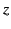. However, let us, for the sake of clarity, imagine that the ionosphere is replaced by a number of thin, discrete, homogeneous, horizontal strata. A continuous ionosphere corresponds to the limit in which the strata become innumerable and infinitely thin. Suppose that a plane wave is incident from below on the ionosphere. Let the wave normal lie in the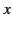-plane, and subtend an angle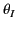with the vertical axis. At the lower boundary of the first stratum, the wave is partially reflected and partially transmitted. The transmitted wave is then partially reflected and partially transmitted at the lower boundary of the second stratum, and so on. However, in the limit of many strata, in which the difference in refractive indices between neighboring strata is very small, the amount of reflection at the boundaries (which is proportional to the square of this difference) becomes negligible. In the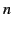th stratum, let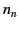be the refractive index, and let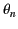be the angle subtended between the wave normal and the vertical axis. According to Snell's law,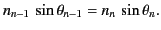(1088)

Below the ionosphere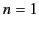, and so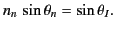(1089)

For an electromagnetic wave in theth stratum, a general field quantity depends onandvia factors of the form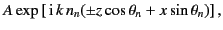(1090)

whereis a constant. The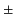signs denote upgoing and downgoing waves, respectively. When the operator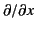acts on the previous expression, it is equivalent to multiplication by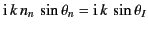, which is independent ofand. It is convenient to use the notation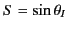. Hence, we may write symbolically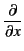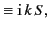(1091)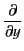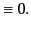(1092)

These results are true no matter how thin the strata are, so they must also hold for a continuous ionosphere. Note that, according to Snell's law, if the wave normal is initially parallel to the-plane then it will remain parallel to this plane as the wave propagates through the ionosphere. Equations (1058)-1061) and (1093)-(1094) can be combined to give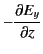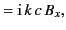(1093)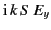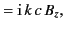(1094)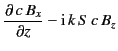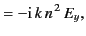(1095)

and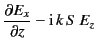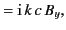(1096)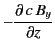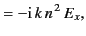(1097)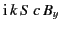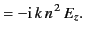(1098)

As before, Maxwell's equations can be split into two independent groups, corresponding to two different polarizations of radio waves propagating through the ionosphere. For the first group of equations, the electric field is always parallel to the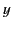-axis. The corresponding waves are, therefore, said to be horizontally polarized. For the second group of equations, the electric field always lies in the-plane. The corresponding waves are, therefore, said to be vertically polarized. (However, the term vertically polarized'' does not necessarily imply that the electric field is parallel to the vertical axis.) Note that the equations governing horizontally polarized waves are not isomorphic to those governing vertically polarized waves. Consequently, both types of waves must be dealt with separately.

For the case of horizontally polarized waves, Equations (1096) and (1097) yield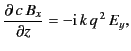(1099)

where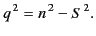(1100)

The previous equation can be combined with Equation (1095) to give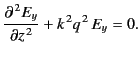(1101)

Equations (1101) and (1103) have exactly the same form as Equations (1064) and (1067), except that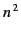is replaced by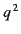. Hence, the analysis of Section 783 can be reused to find the appropriate WKB solution, which take the form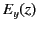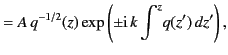(1102)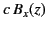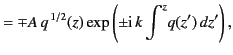(1103)

whereis a constant. Of course, both expressions should also contain a multiplicative factor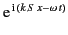, but this is usually omitted for the sake of clarity. By analogy with Equation (1079), the previous WKB solution is valid as long as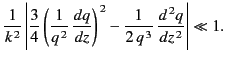(1104)

This inequality clearly fails in the vicinity of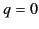, no matter how slowly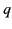varies with. Hence,, which is equivalent to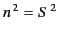, specifies the height at which reflection takes place. By analogy with Equation (1089), the reflection coefficient at ground level (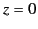) is given by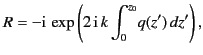(1105)

where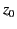is the height at which.

For the case of vertical polarization, Equations (1098) and (1100) yield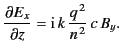(1106)

This equation can be combined with Equation (1099) to give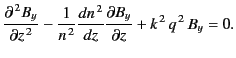(1107)

Clearly, the differential equation that governs the propagation of vertically polarized waves is considerably more complicated than the corresponding equation for horizontally polarized waves.

The WKB solution for vertically polarized waves is obtained by substituting the wave-like solution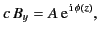(1108)

whereis a constant, and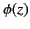is the generalized phase, into Equation (1109). The differential equation thereby obtained for the phase is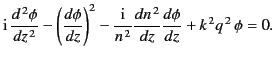(1109)

Because the refractive index is assumed to be slowly varying, the first and third term in the previous equation are small, and so, to a first approximation,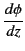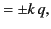(1110)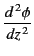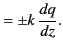(1111)

These expressions can be substituted into the first and third terms of Equation (1111) to give the second approximation,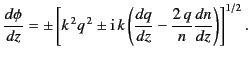(1112)

The final two terms on the right-hand side of the previous equation are small, so expansion of the right-hand side by means of the binomial theorem yields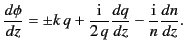(1113)

This expression can be integrated, and the result substituted into Equation (1110), to give the WKB solution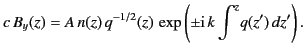(1114)

The corresponding WKB solution for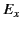is obtained from Equation (1108):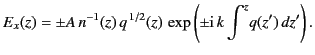(1115)

Here, any terms involving derivatives ofandhave been neglected.

Substituting Equation (1116) into the differential equation (1109), and demanding that the remainder be small compared to the original terms in the equation, yields the following condition for the validity of the previous WKB solution: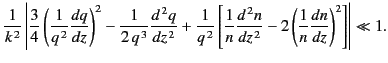(1116)

This criterion fails close to, no matter how slowlyandvary with. Hence,gives the height at which reflection takes place. The condition also fails close to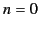, which does not correspond to the reflection level. If, as is usually the case, the electron density in the ionosphere increases monotonically with height then the level at whichlies above the reflection level (where). If the two levels are well separated then the reflection process is unaffected by the failure of the previous inequality at the level, and the reflection coefficient is given by Equation (1107), just as is the case for the horizontal polarization. If, however, the levellies close to the levelthen the reflection coefficient may be affected, and a more accurate treatment of the differential equation (1109) is required in order to obtain the true value of the reflection coefficient.Next: Ionospheric Pulse Propagation Up: Wave Propagation in Inhomogeneous Previous: Reflection Coefficient
Richard Fitzpatrick 2014-06-27